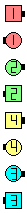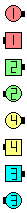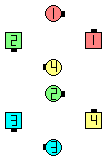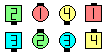Definitions of Square Dance Calls and Concepts
Spin Chain The Line [C3A]Index -->  Plus  |  A1  |  A2  |  C1  |  C2  |  C3A  |  C3B  |  C4  |  NOL  |
Definitions (Text Only) -->  Plus  |  A1  |  A2  |  C1  |  C2  |  C3A  |  C3B  |  C4  |  NOL  |
 Find call:

 \$B8@8l(Bview (admin)

Tidal Wave, Facing Lines, \$B\$^\$?\$OB>\$NE,@Z\$J(B formation \$B\$+\$i!%(B

Arm Turn 1/2 \$B\$r\$7\$F(B, each side \$B\$N(B Centers \$B\$O(B Cast Off 3/4 and Spread \$B\$r\$7(B, Very Centers Trade, Very Ends \$B\$H(B Very Centers \$B\$O6aIU\$\$\$F(B Cast Off 3/4 \$B\$r\$7\$^\$9!%(B

\$BJ?9T\$J(B Line \$B\$G=*\$o\$j\$^\$9!%(BSpin Chain The Line\$B\$NA0(B Arm Turn 1/2\$B\$N8e(B after each side \$B\$N(B Centers \$B\$O(B Cast Off 3/4 \$B\$H(B Spread as Very Centers Trade after Very Ends \$B\$H(B Very Centers \$B\$O6aIU\$\$\$F(B & Cast Off 3/4 (\$B=*\$o\$j(B)

\$BCm

• Very Ends \$B\$H(B Very Centers \$B\$O(B \$B:G8e\$N(B Cast Off 3/4 \$B\$N\$?\$a\$K6aIU\$/\$H\$-(B, each side \$B\$N(B centers \$B\$,%3!<%k\$r=*\$(\$k\$^\$GBT\$C\$F\$\$\$J\$1\$l\$P\$J\$j\$^\$;\$s!%(B
• \$B;d\$?\$A\$,CN\$C\$F\$\$\$k\$b\$C\$H\$b0lHLE*\$J4V0c\$\$\$O(B, \$B;O\$a\$N(B Arm Turn 1/2 \$B\$G(B Very Ends \$B\$H\$J\$C\$??M\$,2?\$r\$7\$?\$i\$\$\$\$\$+J,\$+\$i\$J\$/\$J\$k\$3\$H\$G\$9!%(BVery ends \$B\$K\$J\$C\$?\$i(B, \$B2?%S!<%H\$+\$=\$N>l\$GBT\$C\$F\$\$\$k\$H(B, \$B:G8e\$N(B Cast Off 3/4 \$B\$r\$9\$k(B Very Centers \$B\$N(B 1 \$B?M\$,\$d\$C\$F\$-\$^\$9!%%3!<%k\$G0lHVFq\$7\$\$\$3\$H\$O(B, \$B2?\$+5/\$3\$k\$^\$GBT\$C\$F\$\$\$k\$H\$\$\$&\$3\$H\$G\$9!%(B
• Spin Chain The Line But anything [C3AV]: Resulting Centers replace the final Cast Off 3/4 with the anything call.

Fan Chain The Line [C3B]: Tidal Line \$B\$^\$?\$O(B Facing Lines (\$B\$3\$N>l9g(B, \$B\$^\$:A0?J\$7\$F1&\$N(B Tidal Wave \$B\$r:n\$j\$^\$9(B). Each side \$B\$N(B Centers \$B\$O(B Cast Off 3/4 and Spread \$B\$r\$7(B, Very Centers Trade, Very Ends \$B\$H(B Very Centers \$B\$O6aIU\$\$\$F(B Cast Off 3/4 \$B\$r\$7\$^\$9!%J?9T\$J(B Line \$B\$G=*\$o\$j\$^\$9!%(BCALLERLAB definition for Spin Chain The LineChoreography for Spin Chain The LineComments? Questions? Suggestions?

 https://www.ceder.net/def/spinchaintheline.php?level=C3A&language=japan24-May-2019 19:55:25Copyright © Vic Ceder.  All Rights Reserved.